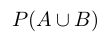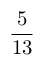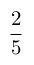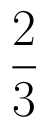Select Page

# Three Dimensional Geometry CBSE Maths 12 Science MCQ Answers in English

Three Dimensional Geometry CBSE Maths 12 Science MCQ Answers in English to enable students to get Answers in a narrative video format for the specific question.

Expert Teacher provides Three Dimensional Geometry CBSE Maths 12 Science MCQ Answers through Video Answers in English language. This video solution will be useful for students to understand how to write an answer in exam in order to score more marks. This teacher uses a narrative style for a question from Three Dimensional Geometry not only to explain the proper method of answering question, but deriving right answer too.

Please find the question below and view the Answer in a narrative video format.

Question:

## Similar Questions from CBSE, 12th Science, Maths, Three Dimensional Geometry

Question 1 : Find the vector equation of the plane with intercepts 3, -4 and 2 on x, y and z-axis respectively. (View Answer Video)

Question 2 : Find the vector equation of the plane which is at a distance of 5 units from the origin and normal to the plane is(View Answer Video)

Question 3 : Find the distance of the point (-1, -5, -10) from the point of intersection of the lineand the plane(View Answer Video)

Question 4 : The cartesian equation of a line is, write its vector form. (View Answer Video)

Question 5 : Write the equation of the straight line through the pointand parallel to z-axis. (View Answer Video)

### Relations and Functions

Question 1 :  If f is the greatest integer function and g is the modulus function . Write the value of g o f(-1/3) – f o g ( -1/3 ) . (View Answer Video)

Question 2 : Considergiven by f(x) = 4x + 3. Find the inverse of f. (View Answer Video)

Question 3 : Let R be the relation on the set N given by R ={(a, b): a = b - 2, b > 6}. Choose the correct answer. (View Answer Video)

Question 4 : A functiondefined as f(x) = 5x is, (View Answer Video)

### Matrices

Question 1 :  Find the value of t, if(View Answer Video)

Question 2 : LetFind A + B. (View Answer Video)

Question 3 : Find the value of x, if. (View Answer Video)

Question 4 : Using elementary transformation, find the inverse of the matrix. (View Answer Video)

Question 5 : Find the value of c from the equation:(View Answer Video)

### Probability

Question 1 : Evaluate, if 2P(A)=P(B)=and=. (View Answer Video)

Question 2 :  A coin is tossed three times. Find P(F/E), where E : at most two tails and F : atleast one tail.  (View Answer Video)

Question 3 :  In a group of 400 people, 160 are smokers and non-vegetarian, 100 are smokers and vegetarian and the remaining are non-smokers and vegetarian. The probabilities of getting a special chest disease are 35%, 20% and 10% respectively. A person is chosen from the group at random and is found to be suffering from the disease. What is the probability that the selected person is a smoker and non-vegetarian? What value is reflected in this question?     (View Answer Video)

Question 4 : A problem in mathematics is given to 4 students A, B, C, D. Their chances of solving the problem, respectively, areand. What is the probability that atmost one of them solve the problem?  (View Answer Video)

Question 5 : If each element of a second-order determinant is either zero or one, what is the probability that the value of the determinant is positive?  (View Answer Video)